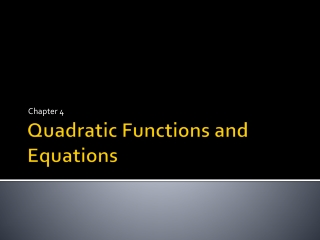DownloadDownload PresentationTélécharger la présentation- - - - - - - - - - - - - - - - - - - - - - - - - - - E N D - - - - - - - - - - - - - - - - - - - - - - - - - - -
##### Presentation Transcript

1. Chapter 4 Quadratic Functions and Equations

2. 4.1 Quadratic Functions and Transformations • Pg. 194 – 201 • Obj: Learn how to identify and graph quadratic functions. • F.BF.3, A.CED.1, F.IF.4, F.IF.6

3. 4.1 Quadratic Functions and Transformations • Parabola – the graph of a quadratic function • Quadratic Function – an equation that has a degree 2 • Vertex Form – • Axis of Symmetry – a line that divides the parabola into two mirror images – x=h • Vertex of the Parabola –the intersection of the parabola and its axis of symmetry – (h,k)

4. 4.1 Quadratic Functions and Transformations • Minimum Value – the y-coordinate of the vertex, if a>0 – the parabola opens upward • Maximum Value – the y-coordinate of the vertex, if a <0 – the parabola opens downward • Parent Quadratic Function -

5. 4.1 Quadratic Functions and Transformations • Reflection, Stretch, and Compression • Reflection – a and –a • Stretch – a>1 • Compression – 0<a<1 • Translation of the Parabola • Horizontal – y=(x-h)² - move |h| units • Vertical – y=x²+k – move |k| units • Horizontal and Vertical – y=(x-h)²+k – move |h| units and |k| units

6. 4.2 Standard Form of a Quadratic Function • Pg. 202 – 208 • Obj: Learn how to graph quadratic functions written in standard form. • A.CED.2, F.IF.4, F.IF.6, F.IF.8, F.IF.9

7. 4.2 Standard Form of a Quadratic Function • Standard Form

8. 4.2 Standard Form of a Quadratic Function • Properties of a Quadratic Function • If a>0, the parabola opens upward. If a<0, the parabola opens downward. • The axis of symmetry is x=-b/2a • Vertex (-b/2a, f(-b/2a)) • Y-intercept (0,c)

9. 4.3 Modeling With Quadratic Functions • Pg. 209-214 • Obj: Learn how to model date with quadratic functions. • F.IF.5, F.IF.4

10. 4.4 Factoring Quadratic Expressions • Pg. 216-223 • Obj: Learn how to find common and binomial factors of quadratic expressions and factor special quadratic expressions. • A.SSE.2

11. 4.4 Factoring Quadratic Expressions • Factoring – rewriting an expression as a product of its factors • Greatest Common Factor (GCF) of an Expression – a common factor of the terms in the expression – the common factor with the greatest coefficient and the greatest exponent

12. 4.4 Factoring Quadratic Expressions • Perfect Square Trinomial – a trinomial that is the square of a binomial • Difference of Two Squares -

13. 4.5 Quadratic Equations • Pg. 226-231 • Obj: Learn how to solve quadratic equations by factoring and graphing. • A.CED.1, A.APR.3, A.SSE.1.a

14. 4.5 Quadratic Equations • Zero of a Function – where the graph of a function intersects the x-axis • Zero-Product Property – If ab=0, then a=0 or b=0.

15. 4.6 Completing the Square • Pg. 233 – 239 • Obj: Learn how to solve equations by completing the square and rewrite functions by completing the square. • A.REI.4.b

16. 4.6 Completing the Square • Completing the Square

17. 4.6 Completing the Square • Solving an Equation by Completing the Square • Rewrite the equation in the form x²+bx=c • Complete the square by adding (b/2)² to each side of the equation. • Factor the trinomial • Find square roots • Solve for x

18. 4.7 The Quadratic Formula • Pg. 240-247 • Obj: Learn how to solve quadratic equations using the Quadratic Formula and determine the number of solutions by using the discriminant. • A.REI.4.b